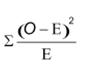# Nursing quiz

##### Nursing Research Quiz - 71 2 3 4 5 6 7 8 9 10 11 12 13 14 15 16 17 18 19 20 21 22 23 24 25 26 27 28 29 30

#### Nursing Research Quiz - 7

##### biostatistics

1. The indices used to measure variation or dispersion among scores are all, except:

A. Range

B. Variance

C. Standard deviation

D. Mean

2. A measure of dispersion of a set of observations in which it is calculated by the difference between the highest and lowest values produced is called:

A. Standard deviation

B. Variance

C. Range

D. Mode

3. A statistic which describes the interval of scores bounded by the 25th and 75th percentile ranks is:

A. Inter quartile range

B. Confidence Interval

C. Standard deviation

D. Variance

4. The Median value is the:

A. 25th percentile

B. 50th percentile

C. 75th percentile

D. 95th percentile

5. Large standard deviations suggest that:

A. scores are probably widely scattered.

B. there is very little deference among scores.

C. mean, median and mode are the same

D. the scores not normally distributed.

6. The formula given below is computational formula for:A. Variance

B. Mean

C. Standard deviation

D. t-statistic

7. The squire of the standard deviation is the:

A. Variance.

B. Standard error

C. Z-score

D. Variance

8. Which is NOT a characteristic of normal distribution?

B. Bell-shaped

C. Mean = median = mode

D. Negative skewness

9. Skewness is a measure:

A. of the asymmetry of the probability distribution

B. decides the distribution may have high or low variance

C. of central tendency

D. None of the above

10. The formula given below is used to calculate:O= Observed frequency E= Expected frequency

A. t-test statistic

B. chi-squire statistic

C. correlation coefficient

D. Standard deviation